﻿ Number Patterns and Sequences
 HOME ABOUT US SHOP PRIVACY CONTACT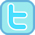# Number Patterns and Sequences (inc. Odd and Even Numbers)

### Free worksheets, interactivities and other resources to support teaching and learning about odd and even numbers and other number sequences and patterns.

#### Number Sequence Printables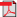Circle the Even Numbers Mad Maths Minutes Sets A & B          Sets C & DCircle the Odd Numbers Mad Maths Minutes Sets A & B          Sets C & D2, 5 and 10 Sequences Mad Maths Minutes A & B Sets A & B          Sets C & DPre-Sequence Work (Addition/Subtraction within 10) Sheet 1          Sheet 2          Sheet 3          Sheet 4Addition Sequences (Numbers to 30, steps up to 5) Sheet 1          Sheet 2          Sheet 3Subtraction Sequences (Numbers to 30, steps up to 5) Sheet 1          Sheet 2          Sheet 3Add/Subtract Sequences (Numbers to 30, steps up to 5) Sheet 1          Sheet 2          Sheet 3Addition Sequences (Numbers to 100, steps up to 10) Sheet 1          Sheet 2          Sheet 3Subtraction Sequences (Numbers to 100, steps up to 10) Sheet 1          Sheet 2          Sheet 3Add/Subtract Sequences (Numbers to 100, steps up to 10) Sheet 1          Sheet 2          Sheet 3Add / Subtract Powers of 10 Sequences Sheet 1          Sheet 2          Sheet 3

#### Interactive Odd and Even ActivitiesOdd or Even Pictures (to 20)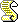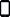Odd or Even (within 20)Odd or Even (within 99)Odd and Even Which Category (to 20)?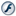Odd and Even Category Click (to 20)Odd Number ShootoutEven Number ShootoutOdd and Even Word Attack#### Interactive Number Sequence Activities (Simple Sequences)Addition Sequences (within 25) - Write the RuleSubtraction Sequences (within 25) Write the RuleAddition / Subtraction Sequences (within 25) Write the RuleAddition Sequences (within 25) What comes next?Subtraction Sequences (within 25) What comes next?Addition / Subtraction Sequences (within 25) What comes next?#### Interactive Number Sequence Activities (Up to 100, steps of up to 10)Addition Sequences (within 100) Write the RuleSubtraction Sequences (within 100) Write the RuleAddition / Subtraction Sequences (within 100) Write the RuleAddition Sequences (within 100)Subtraction Sequences (within 100)Addition / Subtraction Sequences (w. 100)#### Further Sequencing ActivitiesSequences of multiples of 2, 5 or 10 (within 1000)Counting in 25s InteractiveCounting in 1000s InteractiveAdding / Subtracting Powers of 10 Sequences